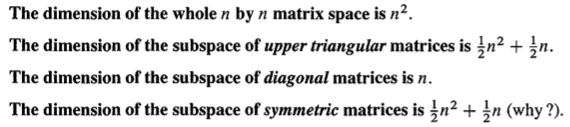Abstract: 本文是本章最重要的知识点，也是整个线性代数中非常核心的内容，包括independence ，basis和dimension等多个概念
Keywords: Independence，Basis，Dimension，Span

# 独立性，基和维度

## 线性独立

Definition: The columns of A are linearly independent when the only solution to $Ax=0$ is $x=0$ No other combination $Ax$ of the columns gives the zero vector

Definition: The sequence of vectors $v_1,\dots,v_n$ is linearly Independence if the only combination that gives the zero vector is $0v_1+0v_2+ \dots +0v_n$

$x_1v_1+x_2v_2+\dots+x_nv_n=0$ only happens when all x’s are zero

## 向量张成子空间（Row Space）

$$v_1=\begin{bmatrix}1\\0\end{bmatrix}\\ v_2=\begin{bmatrix}0\\1\end{bmatrix}$$

$$A=\begin{bmatrix} 1&4\\ 2&7\\ 3&5 \end{bmatrix}\\$$

$$C(A)= x_1 \begin{bmatrix} 1\\ 2\\ 3 \end{bmatrix}+ x_2 \begin{bmatrix} 4\\ 7\\ 5 \end{bmatrix}$$

$$C(A^T)= x_1 \begin{bmatrix}1\\4\end{bmatrix}+x_2 \begin{bmatrix}2\\7\end{bmatrix}+x_3 \begin{bmatrix}3\\5\end{bmatrix}$$

## 基(Basis)

Definition: A basis for a vector space is a sequence of vectors with two properties: The basis vectors are linearty independent and they span the space

Linear combination的性质是所有线性代数知识的合法性来源。

There is one and only one way to write v as a combination of the basis vectors

The columns of the n by n identity Matrix give the “Standard basis” for $\Re^n$

The vectors $v_1,\dots v_n$ are basis for $\Re^n$ exactly when they are the columns of an n by n invertible matrix. Thus $\Re^n$ has infinitely many different bases

The pivot columns of A are a basis for its column space.The pivot rows of A are a basis for its row space . So are the pivot rows of its echelon form R.

If $v_1,\dots,v_m$ and $w_1,\dots,w_n$ are both basis for the same vector space ,then m=n.

## 维度

If $v_1,\dots,v_m$ and $w_1,\dots,w_n$ are both basis for the same vector space ,then m=n.

Definition: The dimension of a space is the number of vectors in every basis.

## 矩阵空间和函数空间(Matrix Space and Function Space00%